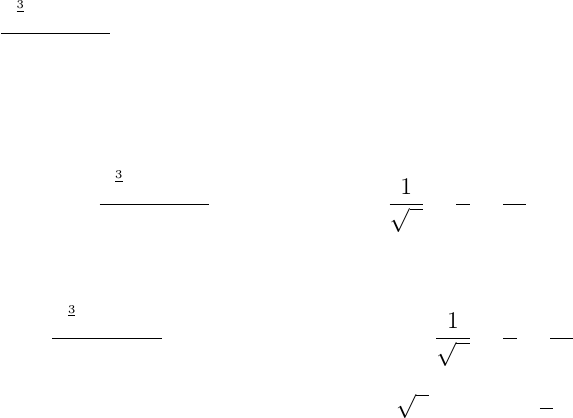Study Guides (390,000)
CA (150,000)
Western (10,000)
MATH (100)
Quiz

# Mathematics 0110A/B Study Guide - Quiz Guide: Antiderivative, Thx, Riemann Sum

Department
Mathematics
Course Code
MATH 0110A/B
Professor
Chris Brandl
Study Guide
Quiz

Page:
of 7MATH 127 Fall 2012
Assignment 9
Topics: Antiderivatives, deﬁnite/indeﬁnite integrals, substitution rule, Riemann sums, and
approximate integration
Due: Wednesday November 28th
Instructions:
Print your name and I.D. number at the top of the ﬁrst page of your solutions, and underline
Submit your solutions in the same order as that of the questions appearing.
Your solutions must have legible handwriting, and must be presented in clear, concise and
logical steps that fully reveal what you are doing.
If you get help or collaborate with someone, then acknowledge the names of those who helped
you. Any outright copying of assignments will be reported as an act of academic plagiarism.
1. Find the following antiderivatives.
(a) Z tan2(x) + x3
2+x+ 2
x2!dx
Since we do not readily know the antiderivative of tan2(x) we use the trig identity
tan2(x) + 1 = sec2(x). Using the identity and simplifying we get
tan2(x) + x3
2+x+ 2
x2= sec2(x)1 + 1
x+1
x+2
x2
From this we see that
Z tan2(x) + x3
2+x+ 2
x2!dx =Zsec2(x)1 + 1
x+1
x+2
x2dx
= tan (x)x+ 2x+ ln |x| − 2
x+C
1
(b) Z1 + sin3(x)
sin2(x)dx
Simplifying we get
1 + sin3(x)
sin2(x)= csc2(x) + sin (x)
From this we see that
Z1 + sin3(x)
sin2(x)dx =Zcsc2(x) + sin (x)dx
=cot (x)cos (x) + C
(c) Zdx
2xx2
First consider the expression 2xx2. If we complete the square we get
2xx2=(x1)2+ 1
So then we have that
1
2xx2=1
p1(x1)2
Let u=x1, we get that du =dx. This substitution gives
Zdx
2xx2=Zdu
1u2
= arcsin (u) + C
= arcsin (x1) + C
(d) Ze2x
ex1dx
Let u=ex1 so then du =exdx. Notice that we can re-write
e2xdx =exexdx = (u+ 1)du
We then get
Ze2x
ex1dx =Zu+ 1
udu
=Z1 + 1
udu
=u+ ln |u|+C
=ex1 + ln |ex1|+C
2
(e) Zcos 1
x
x2dx
Let u=1
xso then du =1
x2dx. We get that
Zcos 1
x
x2dx =Zcos (u)du
=sin (u) + C
=sin 1
x+C
2. Evaluate the following integrals.
(a) Z2
0
(x23)2dx
Z2
0
(x23)2dx =Z2
0
(x46x2+ 9)dx
=x5
56x3
3+ 9x
2
0
=25
52(23) + 9(2) 0
=32
5+ 2
=42
5
(b) Z4
0
x3
x2+ 1dx
Let u=x2+ 1, we get that du = 2xdx. When x= 0 we get u= 1 and when
x= 4 we have u= 17. Notice that x3dx =x2xdx = (u1) 1
2du.
3